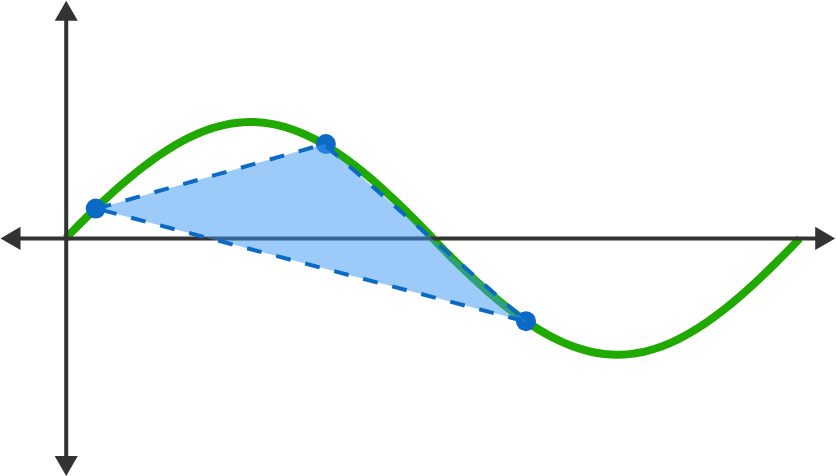# Problems of the Week

Contribute a problem

# 2018-12-03 Advanced

I want to partition the set of positive integers $\{1,2,3,\ldots\}$ into subsets such that each subset satisfies the property that if a number $x$ is in it, $2x$ is not.

What is the minimum possible number of subsets that I can get?

You and some friends would like to explore a labyrinth from its entrance to its exit:

• The labyrinth only has one entrance point and one exit point.
• The labyrinth is located on an infinite square grid; you can only travel in the cardinal directions: East, West, North, and South.
• The labyrinth is infinite in size, but the shortest path from the entrance to the exit is finite. The entrance and exit are both located on the boundaries of the labyrinth.
• You have a communication system that allows one to communicate to the others that the exit has been found (but allows no other communication).

What is the minimum number of people that need to enter the labyrinth for there to be a strategy to guarantee that all can come out at the exit in a finite amount of time?You are given the following information about the number of possible tic-tac-toe games:

• The number of games that end on $N$ moves is $M_{ N }.$
• The number of games that end in draws is $D.$

How many distinct tic-tac-toe games are there?

$62208\big(a^{7}+b^{7}+c^{7}+d^{7}\big)^{2}\le M\big(a^{2}+b^{2}+c^{2}+d^{2}\big)^{7}$

What is the smallest positive integer $M$ such that this inequality holds true for all $a,b,c,d$ satisfying $a,b,c,d\in \mathbb{R}$ and $a+b+c+d=0?$

$x_{1}$, $x_{2}$, and $x_{3}$ are chosen randomly and independently on the interval $[0,2\pi]$ and let $f(x)=\sin(x)$.

What is the average area of the triangle created with the three vertices below (to two significant figures)?

$P_{1}=\big( x_{1} , f( x_{1})\big),\quad P_{2}=\big( x_{2} , f( x_{2})\big),\quad P_{3}=( x_{3} , f( x_{3})\big)$×

Problem Loading...

Note Loading...

Set Loading...